## Plan for Today

• Graphics
• Two variables
• Formulas
• Linear Regression
• R programs

plot(birth$weight)each individual has a position in the x axis ## Ploting Factors plot(birth$sex)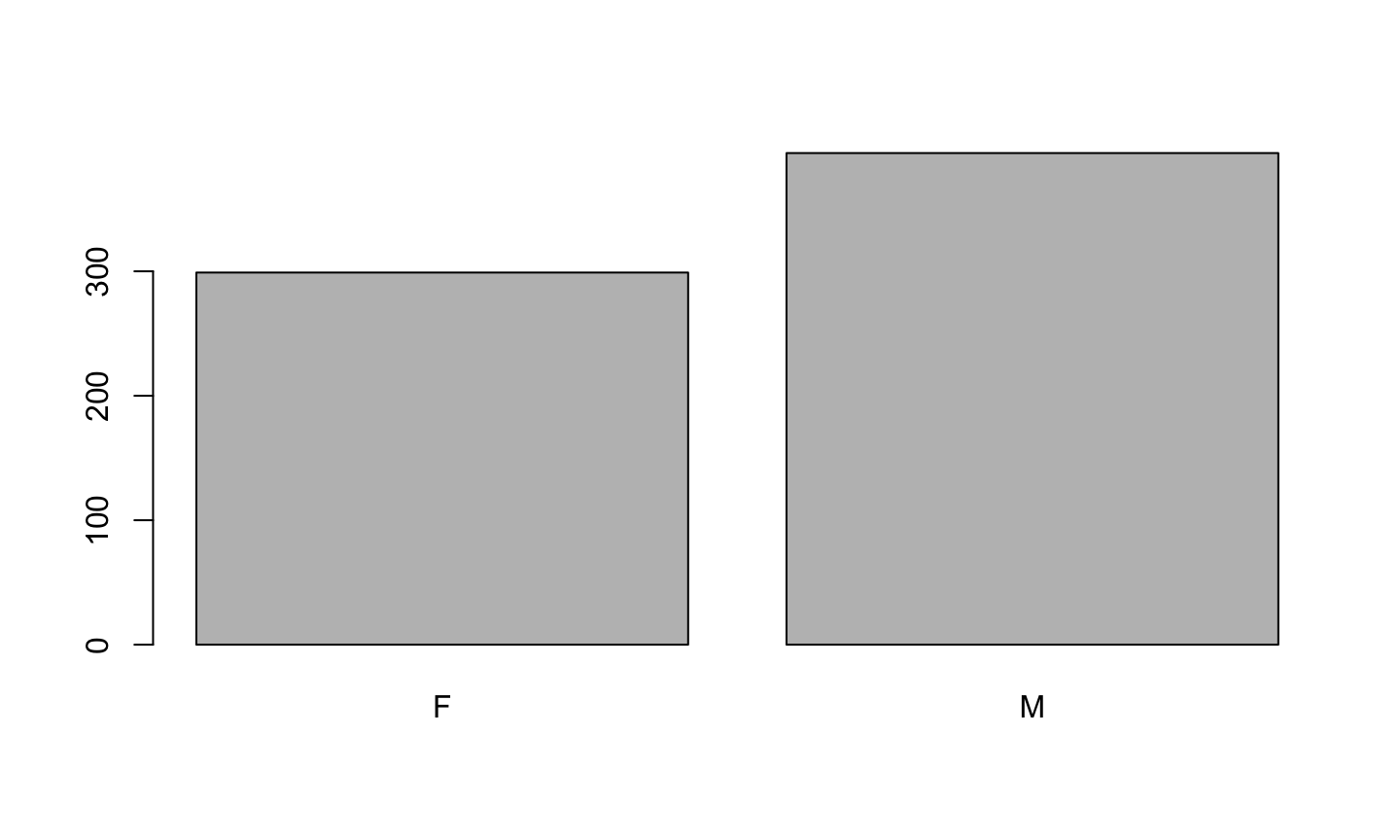## Histograms

par(mfrow = c(1,2))
plot(birth$head) hist(birth$head, col="grey", nclass = 30)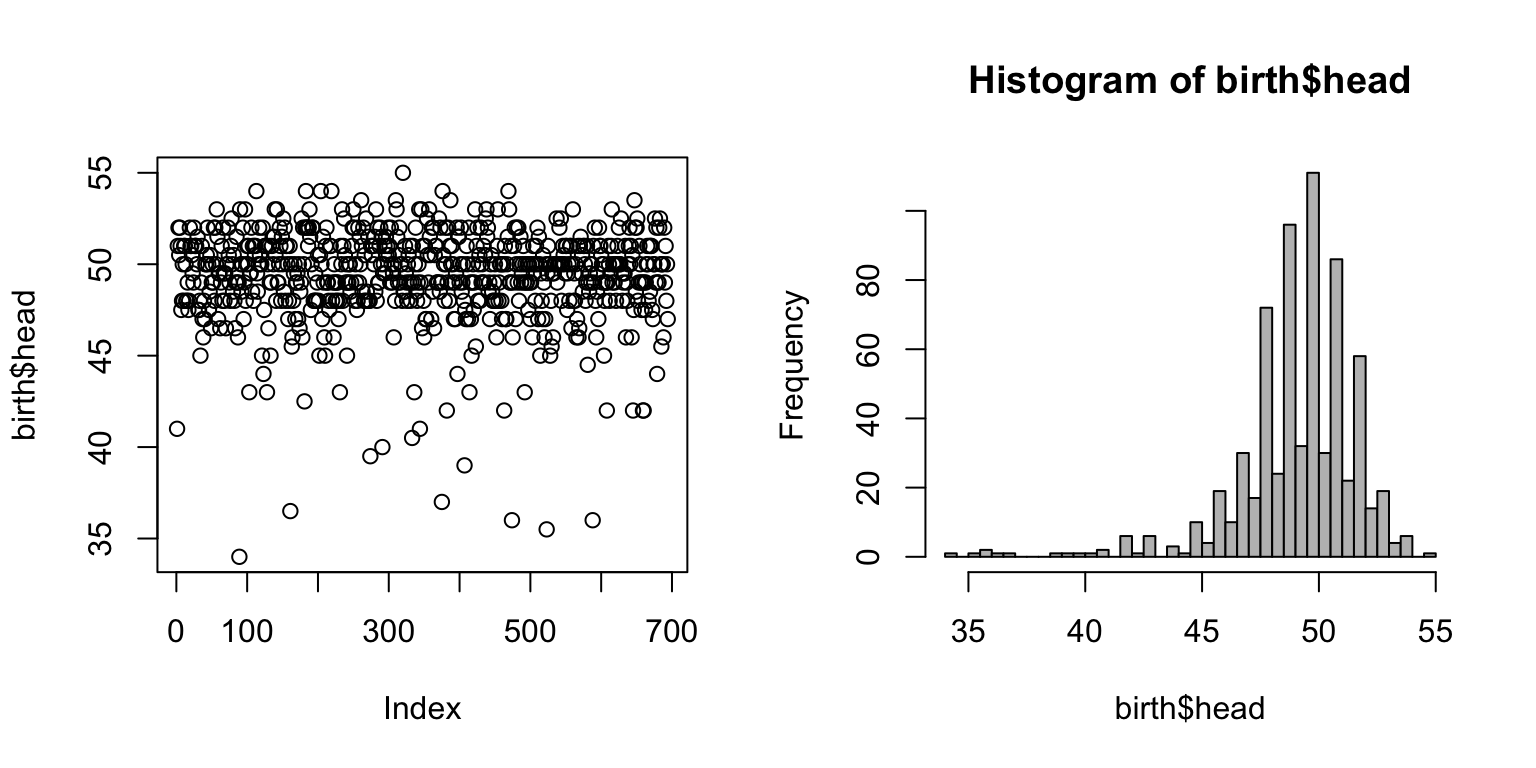## Chosing the color

par(mfrow = c(1,2))
plot(birth$head) plot(birth$head, col="red")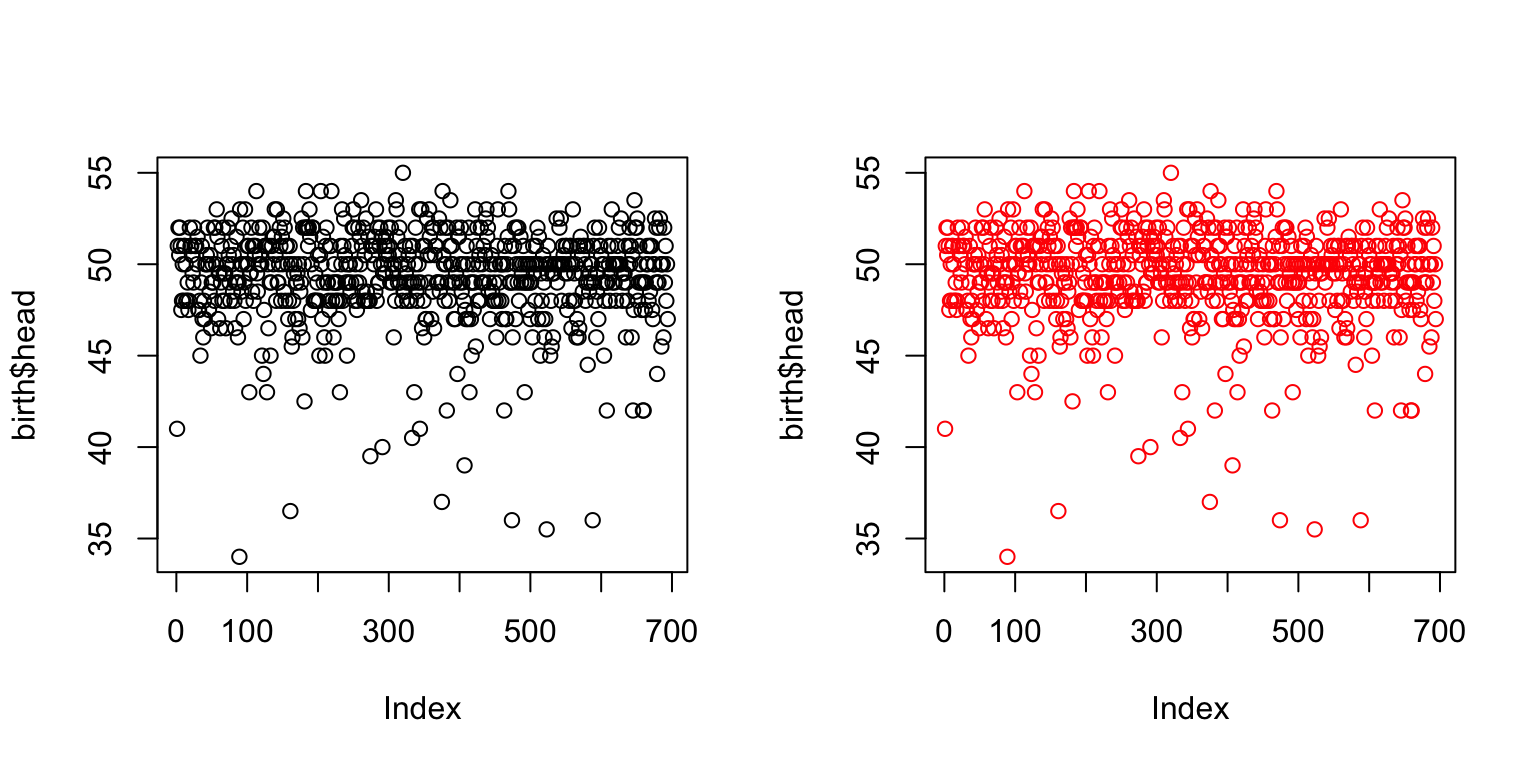## Chosing the size of the symbol

par(mfrow = c(1,2))
plot(birth$head, cex=2) plot(birth$head, cex=0.5)## Chosing the symbol

par(mfrow = c(1,2))
plot(birth$head, pch=16) plot(birth$head, pch=".")## Chosing the type of plot

par(mfrow = c(1,2))
plot(birth$head, type = "l") plot(birth$head, type = "o")## Zooming

par(mfrow = c(1,2))
plot(birth$head, type = "l", xlim=c(1,100)) plot(birth$head, type = "o", xlim=c(1,100))lines(birth$age, col="red")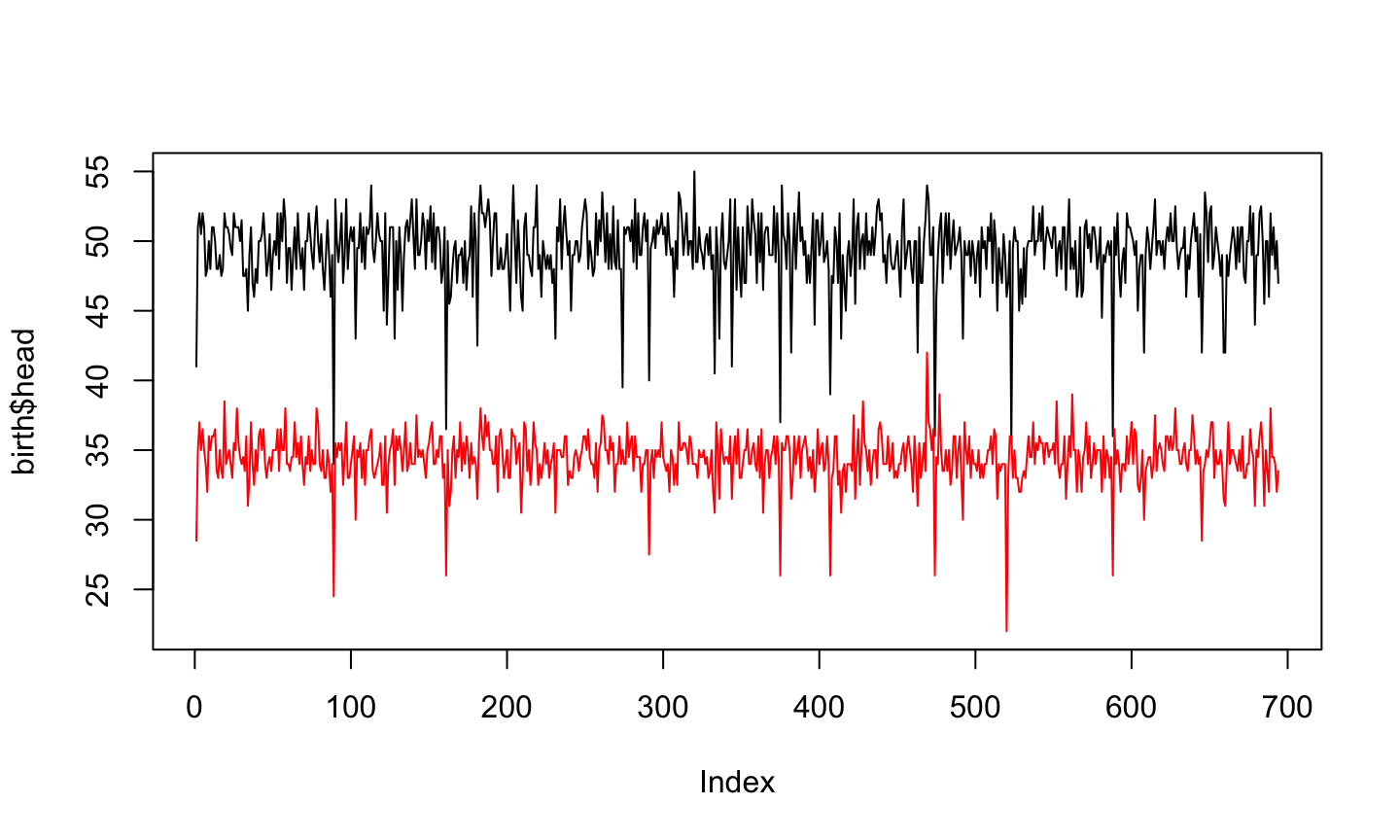## Boys and Girls plot(birth$weight[birth$sex=="M"], ylim=range(birth$weight), ylab = "weight [gr]")
points(birth$weight[birth$sex=="F"], col="blue")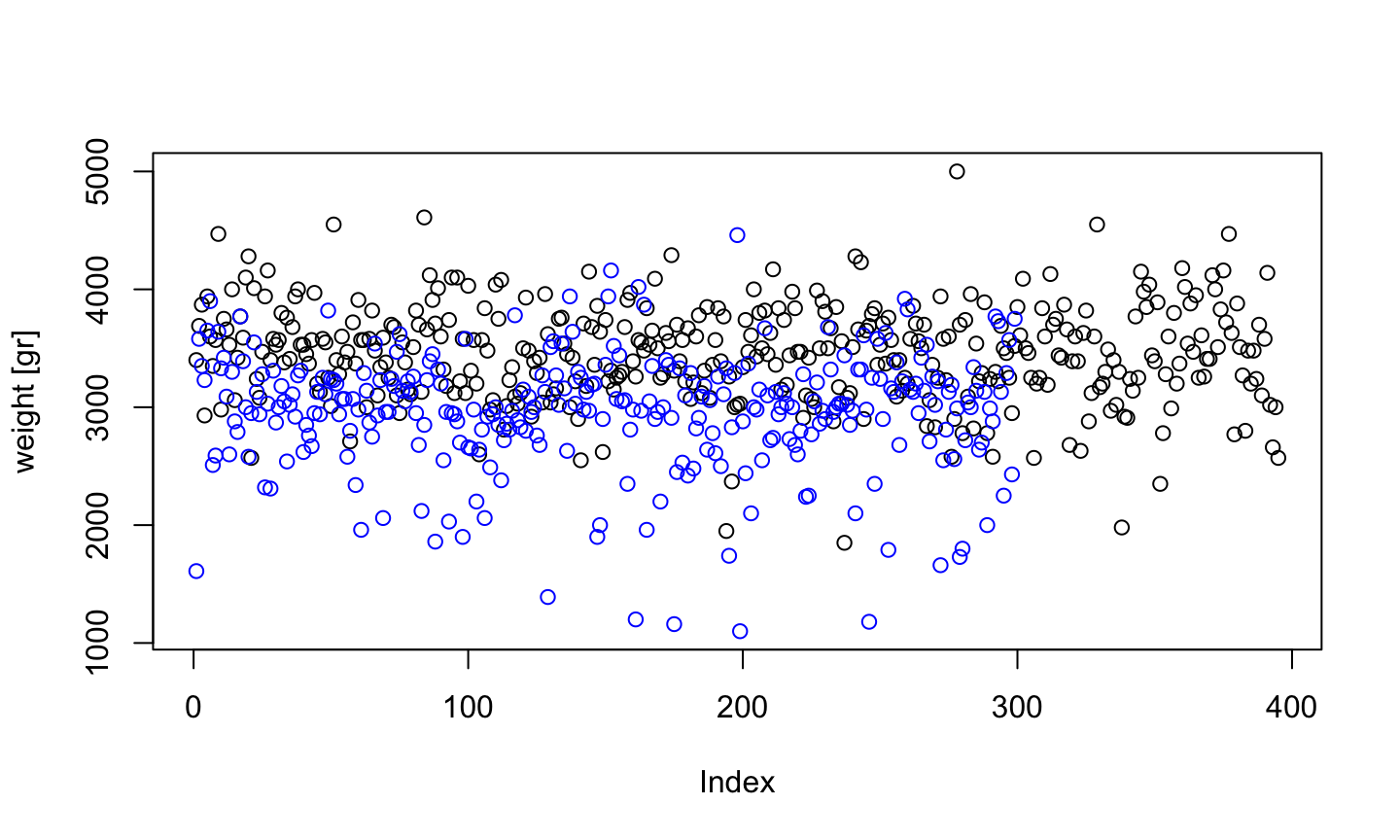## Using auxiliary variables

boys <- birth$weight[birth$sex=="M"]
girls <- birth$weight[birth$sex=="F"]
plot(boys, ylim=range(birth$weight), ylab = "weight [gr]") points(girls, col="blue") abline(h=median(boys)) abline(h=median(girls), col="blue") legend("topright", c("boys","girls"), fill=c("black","blue"))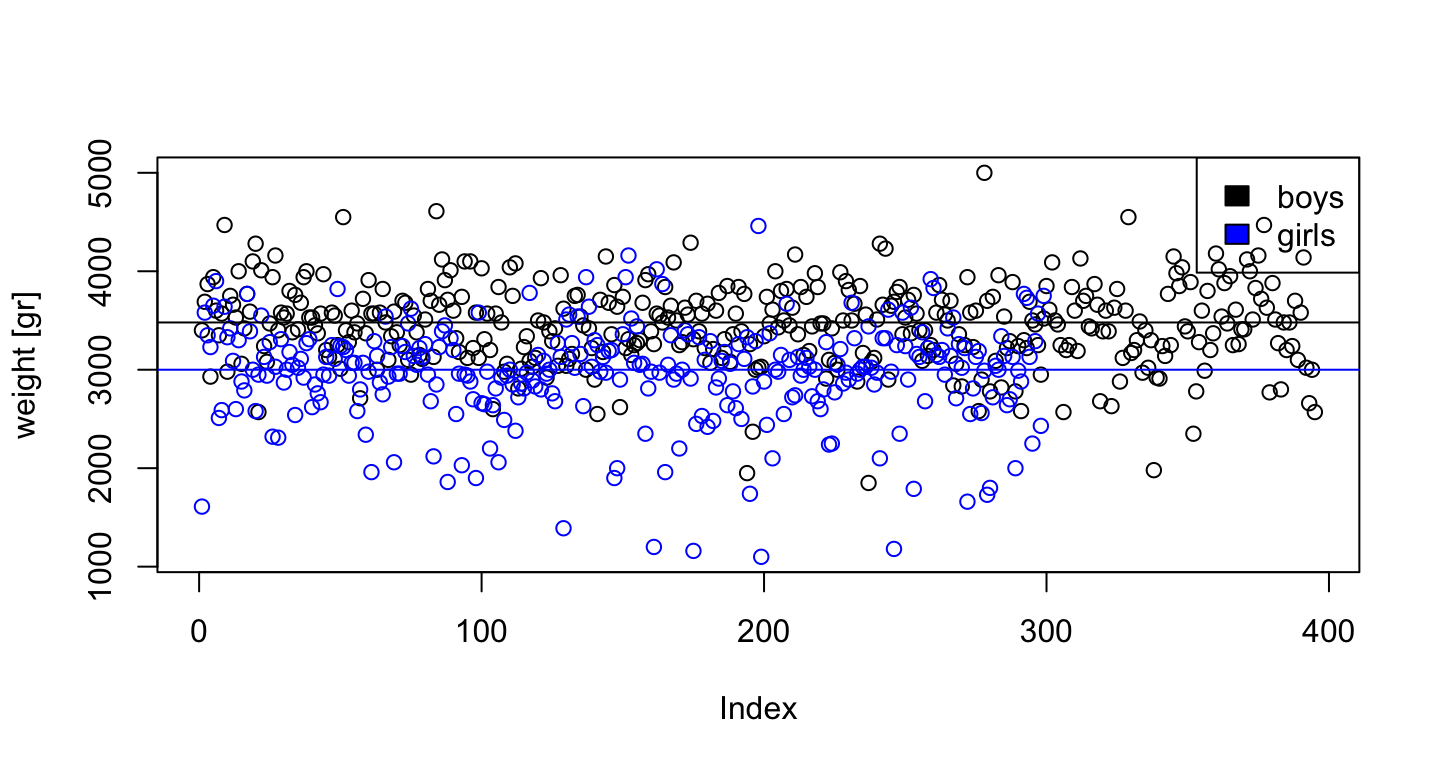## abline This command adds a straigth line in a specific position • abline(h=1) adds a horizontal line in 1 • abline(v=2) adds a vertical line in 2 • abline(a=3, b=4) adds an $$a +b\cdot x$$ line ## Scatter plots ## Comparing two variables plot(birth$age, birth$apgar5)## Add jitter to see more plot(jitter(birth$age), jitter(birth\$apgar5))Less precise but more informative